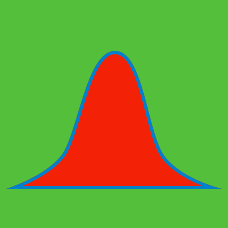Probability

# Normal Distribution

If $X$ is a normally distributed variable with mean $\mu = 11$ and standard deviation $\sigma = 5,$ then what is the probability $P(X > 21)?$

Note: Use the standard normal distribution table below, where $Z$ has mean $\mu = 0$ and standard deviation $\sigma = 1.$

$\begin{matrix} z & P(0 \leq Z \leq z) \\ 1 & 0.3413 \\ 1.5 & 0.4332 \\ 2.0 & 0.4772 \\ 2.5 & 0.4938 \end{matrix}$

If $X$ is a normally distributed variable with mean $\mu = 13$ and standard deviation $\sigma = 4,$ then what is the probability $P(13

Note: Use the following normal distribution table, where $Z$ is standardization of $X$ with $\mu = 0$ and $\sigma = 1:$

$\begin{matrix} z & P(0 \leq Z \leq z) \\ 1 & 0.3413 \\ 1.5 & 0.4332 \\ 2.0 & 0.4772 \\ 2.5 & 0.4938 \end{matrix}$

A large group of students took a math test, and their scores obey a normal distribution. If the distribution has mean $62$ and standard deviation $10,$ what is the percentage of those students who scored higher than $72?$

Note: Use the standard normal distribution table below, where $Z$ has mean $\mu = 0$ and standard deviation $\sigma = 1.$

$\begin{matrix} z & P(0 \leq Z \leq z) \\ 1 & 0.3413 \\ 1.5 & 0.4332 \\ 2.0 & 0.4772 \\ 2.5 & 0.4938 \end{matrix}$

There are a total of 400 students in a secondary school. Their heights obey a normal distribution with mean $169 \text{ cm}$ and standard deviation $4 \text{ cm}.$ What is the approximate number of students whose heights are below $163 \text{ cm}$ or above $171 \text{ cm}?$

Note: Use the standard normal distribution table below, where $Z$ has mean $\mu = 0$ and standard deviation $\sigma = 1.$

$\begin{matrix} z & P(0 \leq Z \leq z) \\ 0.5 & 0.1915 \\ 1 & 0.3413 \\ 1.5 & 0.4332 \\ 2.0 & 0.4772 \\ \end{matrix}$

If $X$ is a normally distributed variable with mean $\mu = 16$ and standard deviation $\sigma = 4,$ then what is the probability $P(X<26 )?$

Note: Use the standard normal distribution table below, where $Z$ has mean $\mu = 0$ and standard deviation $\sigma = 1.$

$\begin{matrix} z & P(0 \leq Z \leq z) \\ 1 & 0.3413 \\ 1.5 & 0.4332 \\ 2.0 & 0.4772 \\ 2.5 & 0.4938 \end{matrix}$

×